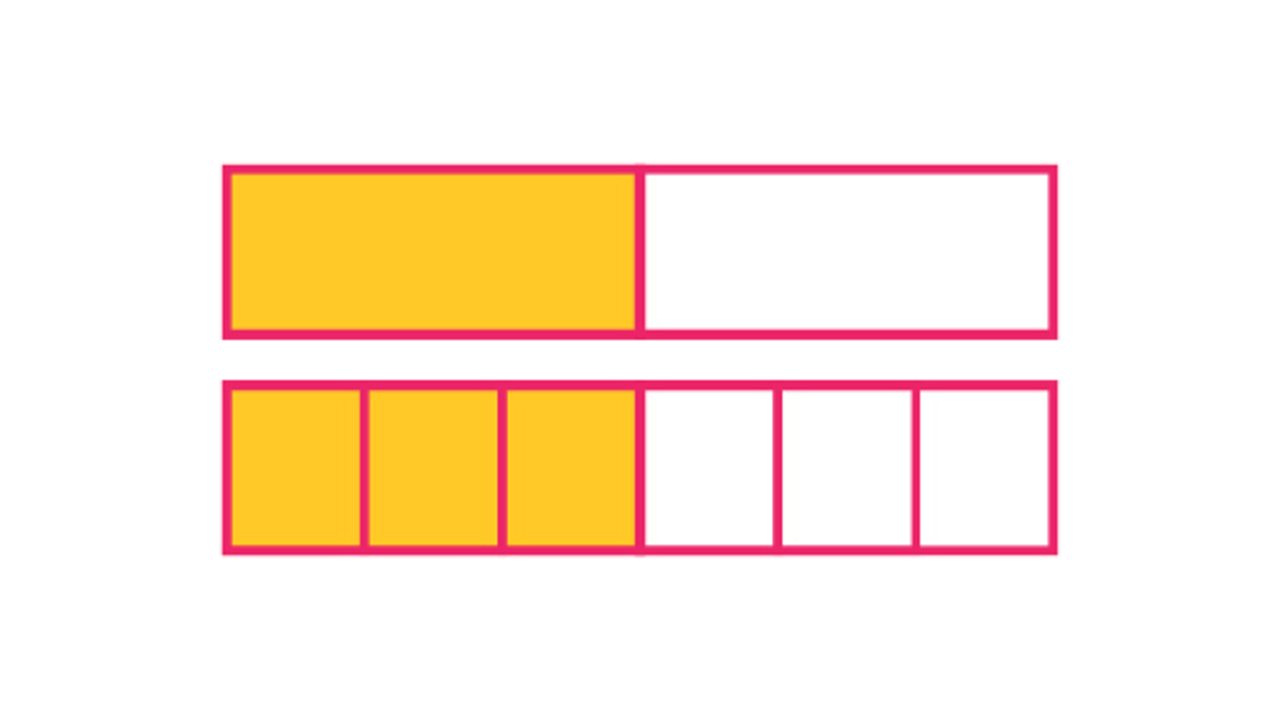# What are equivalent fractions?

• Sometimes fractions can be simplified into smaller ones.

• These fractions are said to be equivalent.

• You can multiply the numerator and denominator in the smaller fraction, by the same number, and get the first fraction back.

## Simplifying fractions

When working with a fraction, it is much easier to use it in its simplest form. You can simplify a fraction by dividing the numerator and denominator by the same number.

You won't be able to simplify a fraction if the same number won't divide into both the numerator and denominator. The simplified fraction and the original fraction are said to be equivalent.

Other examples of equivalent fraction pairs include:

## Visualising fractions

Equivalent fractions can best be visualised by using a bar model. If you take two identical bars and split one in half and the other into sixths you will see that one half is exactly the same as three sixths, i.e. ¹/₂ = ³/₆.

This works because when 1 is the numerator and 2 is denominator of a fraction, and both are multiplied by 3, you get 3 and 6 (or three-sixths).½ equals ³/₆